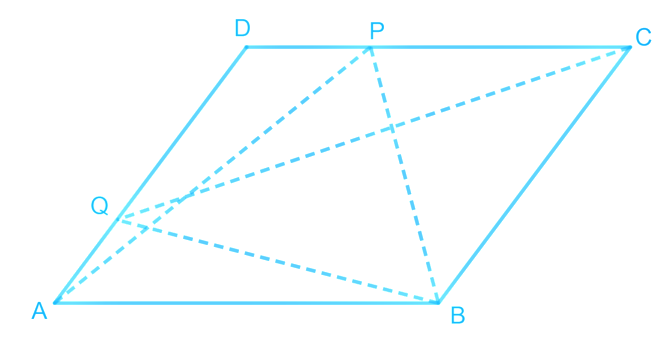# Ex.9.2 Q3 Areas of Parallelograms and Triangles Solution - NCERT Maths Class 9

Go back to  'Ex.9.2'

## Question

$$P$$ and $$Q$$ are any two points lying on the sides $$DC$$ and $$AD$$ respectively of a parallelogram $$ABCD$$. Show that $$ar (APB) = ar (BQC)$$.

Video Solution
Areas Of Parallelograms And Triangles
Ex 9.2 | Question 3

## Text Solution

What is known?

$$P$$ and $$Q$$ are any two points lying on the sides $$DC$$ and $$AD$$ respectively of a parallelogram $$ABCD$$.

What is unknown?

How we can show that $$ar (APB) = ar (BQC).$$

Reasoning:

If a triangle and parallelogram are on same base and between same parallel lines, then area of triangle will be half of area of parallelogram. So, we can find parallelogram area by two ways and equate them to get the required result.

Steps:It can be observed that $$\Delta BQC$$ and parallelogram $$ABCD$$ lie on the same base $$BC$$ and these are between the same set of parallel lines $$AD$$ and $$BC$$.

\begin{align}\therefore \rm{Area} \left( \Delta \,{BQC} \right)\!\!=\!\!\frac{1}{2}\text{Area }\!\left( {ABCD} \right)\!\!\ldots \left( 1 \right)\end{align}

Similarly, $$\Delta APB$$ and parallelogram $$ABCD$$ lie on the same base $$AB$$ and between the same set of parallel lines $$AB$$ and $$DC$$.

\begin{align}\therefore\!\text { Area }\!(\Delta {APB})\!\!=\!\!\frac{1}{2} \text { Area }\!({ABCD}) \!\cdots(2)\end{align}

From Equations ($$1$$) and ($$2$$), we obtain \begin{align}\rm {Area}\; (\Delta BQC) = Area\; (\Delta APB)\end{align}

Video Solution
Areas Of Parallelograms And Triangles
Ex 9.2 | Question 3

Learn from the best math teachers and top your exams

• Live one on one classroom and doubt clearing
• Practice worksheets in and after class for conceptual clarity
• Personalized curriculum to keep up with school